# Checking a Poisson distribution fit: An example with officer involved shooting deaths WaPo data (R functions)

So besides code on my GitHub page, I have a list of various statistic functions I’ve scripted on the blog over the years on my code snippets page. One of those functions I will illustrate today is some R code to check the fit of the Poisson distribution. Many of my crime analysis examples rely on crime data being approximately Poisson distributed. Additionally it is relevant in regression model building, e.g. should I use a Poisson GLM or do I need to use some type of zero-inflated model?

Here is a brief example to show how my R code works. You can source it directly from my dropbox page. Then I generated 10k simulated rows of Poisson data with a mean of 0.2. So I see many people in CJ make the mistake that, OK my data has 85% zeroes, I need to use some sort of zero-inflated model. If you are working with very small spatial/temporal units of analysis and/or rare crimes, it may be the mean of the distribution is quite low, and so the Poisson distribution is actually quite close.

``````# My check Poisson function
source('https://dl.dropboxusercontent.com/s/yj7yc07s5fgkirz/CheckPoisson.R?dl=0')

# Example with simulated data
set.seed(10)
lambda <- 0.2
x <- rpois(10000,lambda)
CheckPoisson(x,0,max(x),mean(x))``````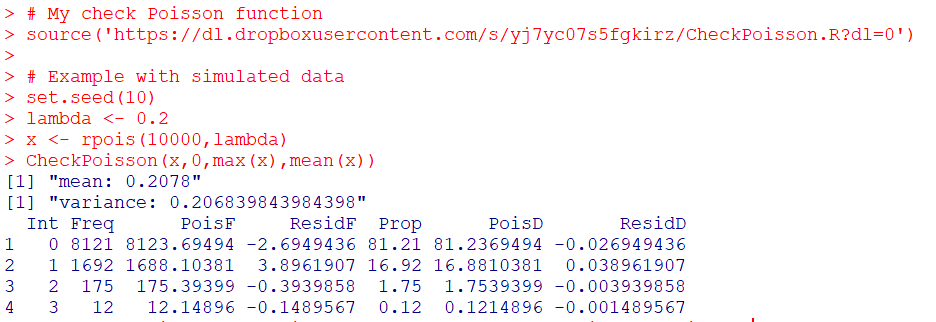Here you can see in the generated table from my `CheckPoisson` function, that with a mean of 0.2, we expect around 81.2% zeroes in the data. And since we simulated the data according to the Poisson distribution, that is what we get. The table shows that out of the 10k simulation rows, 8121 were 0’s, 1692 rows were 1’s etc.

In real life data never exactly conform to hypothetical distributions. But we often want to see how close they are to the hypothetical before building predictive models. A real life example as close to Poisson distributed data as I have ever seen is the Washington Post Fatal Use of Force data. Every year WaPo has been collating the data, the total number of Fatal uses of Police Force in the US have been very close to 1000 events per year. And even in all the turmoil this past year, that is still the case.

``````# Washington Post Officer Involved Shooting Deaths Data
stringsAsFactors = F)

# Year Stats
oid\$year <- as.integer(substr(oid\$date,1,4))
year_stats <- table(oid\$year)[1:6]
year_stats
mean(year_stats)
var(year_stats)``````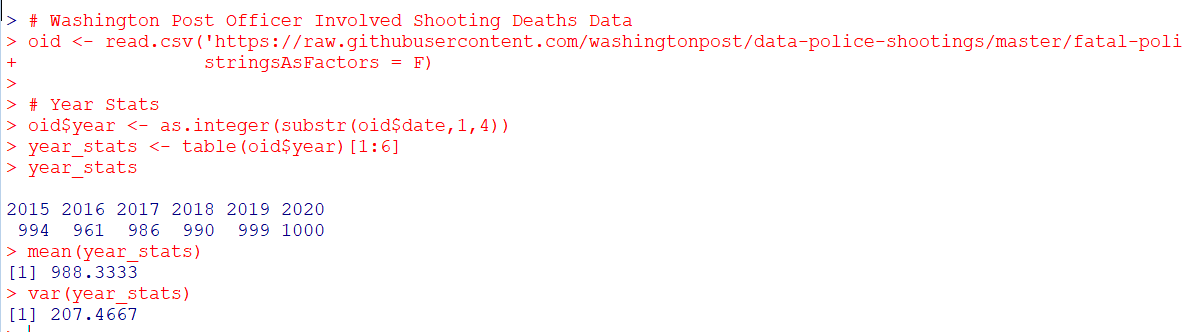One way to check the Poison distribution is that the mean and the variance should be close, and here at the yearly level the data have some evidence of underdispersion according to the Poisson distribution (most crime data is overdispersed – the variance is much greater than the mean). If the actual mean is around 990, you would expect typical variations of say around plus/minus 60 per year (`~ 2*sqrt(990)`). But that only gives us a few observations to check (6 years). We can dis-aggregate the data to smaller intervals and check the Poisson assumption. Here I aggregate to days (note that this includes zero days in the table levels calculation). Then we again check the fit of the Poisson distribution.

``````#Now aggregating to count per day
oid\$date_val <- as.Date(oid\$date)
date_range <- paste0(seq(as.Date('2015-01-01'),max(oid\$date_val),by='days'))
day_counts <- as.data.frame(table(factor(oid\$date,levels=date_range)))
pfit <- CheckPoisson(day_counts\$Freq, 0, 10, mean(day_counts\$Freq))
pfit``````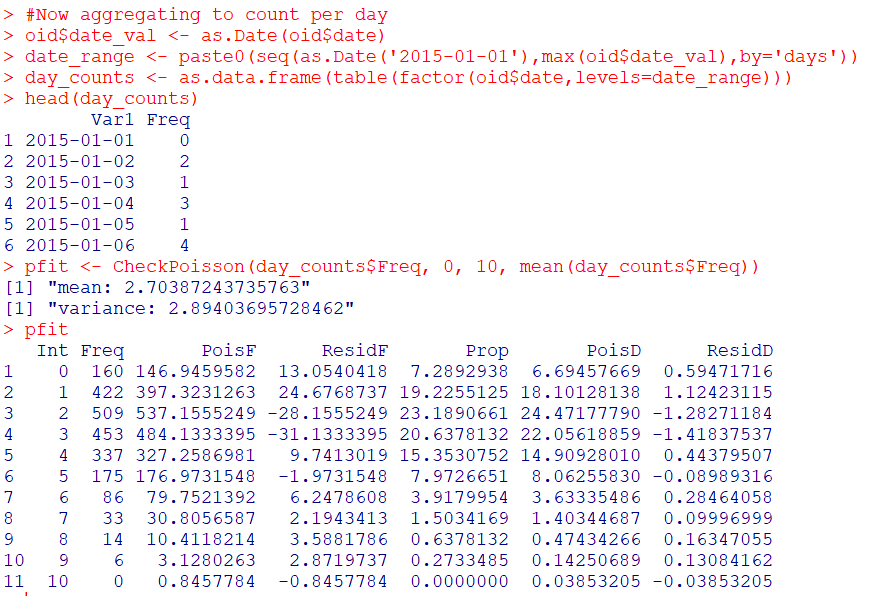According to the mean and the variance, it appears the distribution is a very close fit to the Poisson. We can see in this data we expected to have around 147 days with 0 fatal encounters, and in reality there were 160. I like seeing the overall counts, but another way is via the proportions in the final three columns of the table. You can see for all of the integers, we are less than 2 percentage points off for any particular integer count. E.g. we expect the distribution to have 3 fatal uses of force on about 22% of the days, but in the observed distribution days with 3 events only happened around 21% of the days (or `20.6378132` without rounding). So overall these fatal use of force data of course are not exactly Poisson distributed, but they are quite close.

So the Poisson distribution is motivated via a process in which the inter-arrival dates of events being counted are independent. Or in more simple terms one event does not cause a future event to come faster or slower. So offhand if you had a hypothesis that publicizing officer fatalities made future officers more hesitant to use deadly force, this is not supported in this data. Given that this is officer involved fatal encounters in the entire US, it is consistent with the data generating process that a fatal encounter in one jurisdiction has little to do with fatal encounters in other jurisdictions.

(Crime data we are often interested in the opposite self-exciting hypothesis, that one event causes another to happen in the near future. Self-excitation would cause an increase in the variance, so the opposite process would result in a reduced variance of the counts. E.g. if you have something that occurs at a regular monthly interval, the counts of that event will be underdispersed according to a Poisson process.)

So the above examples just checked a univariate data source for whether the Poisson distribution was a decent fit. Oftentimes academics are interested in whether the conditional distribution is a good fit post some regression model. So even if the marginal distribution is not Poisson, it may be you can still use a Poisson GLM, generate good predictions, and the conditional model is a good fit for the Poisson distribution. (That being said, you model has to do more work the further away it is from the hypothetical distribution, so if the marginal is very clearly off from Poisson a Poisson GLM probably won’t fit very well.)

My `CheckPoisson` function allows you to check the fit of a Poisson GLM by piping in varying predicted values over the sample instead of just one. Here is an example where I use a Poisson GLM to generate estimates conditional on the day of the week (just for illustration, I don’t have any obvious reason fatal encounters would occur more or less often during particular days of the week).

``````#Do example for the day of the week
day_counts\$wd <- weekdays(as.Date(day_counts\$Var1))
mod <- glm(Freq ~ as.factor(wd) - 1, family="poisson", data=day_counts)
#summary(mod), Tue/Wed/Thu a bit higher
lin_pred <- exp(predict(mod))
pfit_wd <- CheckPoisson(day_counts\$Freq, 0, 10, lin_pred)
pfit_wd``````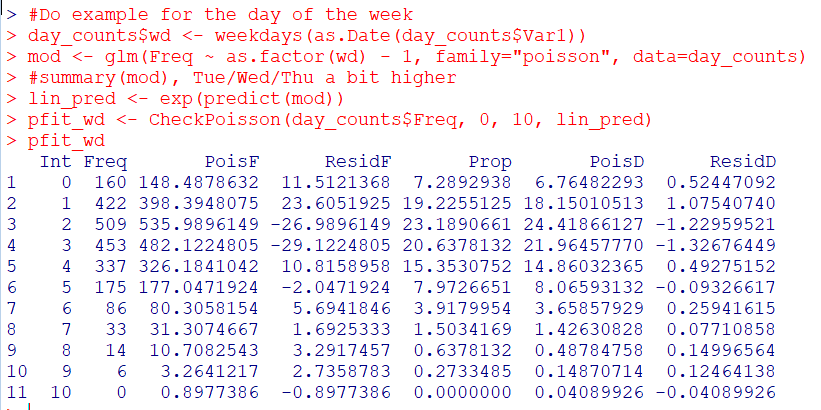You can see that the fit is almost exactly the same as before with the univariate data, so the differences in days of the week does not explain most of the divergence from the hypothetical Poisson distribution, but again this data is already quite close to a Poisson distribution.

So it is common for people to do tests for goodness-of-fit using these tables. I don’t really recommend it – just look at the table and see if it is close. Departures from hypothetical can inform modeling decisions, e.g. if you do have more zeroes than expected than you may need a negative binomial model or a zero-inflated model. If the departures are not dramatic, variance estimates from the Poisson assumption are not likely to be dramatically off-the-mark.

But if you must, here is an example of generating a Chi-Square goodness-of-fit test with the example Poisson fit table.

``````# If you really want to do a test of fit
chi_stat <- sum((pfit\$Freq - pfit\$PoisF)^2/pfit\$PoisF)
df <- length(pfit\$Freq) - 2
dchisq(chi_stat, df)``````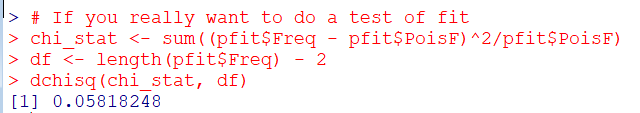So you can see in this example the p-value is just under 0.06.

I really don’t recommend this though for two reasons. One is that with null hypothesis significance testing you are really put in a position that large data samples always reject the null, even if the departures are trivial in terms of the assumptions you are making for whatever subsequent model. The flipside of this is that with small samples the test is underpowered, so there are never many good scenarios where it is useful in practice. Two, you can generate superfluous categories (or collapse particular categories) in the Chi-Square test to increase the degrees of freedom and change the p-value.

One of the things though that this is useful for is checking the opposite, people fudging data. If you have data too close to the hypothetical distribution (so very high p-values here), it can be evidence that someone manipulated the data (because real data is never that close to hypothetical distributions). A famous example of this type of test is whether Mendel manipulated his data.

I intentionally chose the WaPo data as it is one of the few that out of the box really appears to be close to Poisson distributed in the wild. One of my next tasks though is to do some similar code for negative binomial fits. Like Paul Allison, for crime count data I rarely see much need for zero-inflated models. But while I was working on that I noticed that the parameters in NB fits with even samples of 1,000 to 10,000 observations were not very good. So I will need to dig into that more as well.

1.#### Gio

/  January 13, 2021

This is a fairly interesting question. You can directly test a lot of different distributions easily using Stan in either Python or R. Here I just fit a model for the mean using different distributions in rstanarm then check the fit using `pp_check`.

Model Fits: https://imgur.com/a/CjsTyJ5

The Gaussian is clearly inappropriate, but the distinctions between a Poisson and Neg Binomial are a lot more subtle. A pure Poisson model tends to over-predict days with 2-3 shootings, while a Neg Binomial seems a bit more tightly fit around that range despite over-predicting days with zero shootings. If I was actually fitting a real model I would probably tweak the priors on the Neg Binomial model too, as a few iterations are a bit extreme.

Code:

library(tidyverse)
library(rstanarm)
library(lubridate)

# Washington Post Officer Involved Shooting Deaths Data
stringsAsFactors = F)

date_grid <- cbind.data.frame(date = seq.Date(from = ymd("2015-01-01"), to = ymd("2020-12-31"), by = "day"))

oid_deaths %
mutate(date = as_date(date)) %>%
count(date) %>%
right_join(date_grid) %>%
replace_na(list(n = 0)) %>%
arrange(date)

# Check distribution of deaths
hist(oid_deaths\$n)
mean(oid_deaths\$n)
var(oid_deaths\$n)

# Fits
fit1 <- stan_glm(n ~ 1, data = oid_deaths, family = gaussian())
fit2 <- stan_glm(n ~ 1, data = oid_deaths, family = poisson())
fit3 <- stan_glm(n ~ 1, data = oid_deaths, family = neg_binomial_2())

pp_check(fit1) + labs(title = "Gaussian")
pp_check(fit2) + labs(title = "Poisson")
pp_check(fit3) + labs(title = "Neg. Binomial)

•